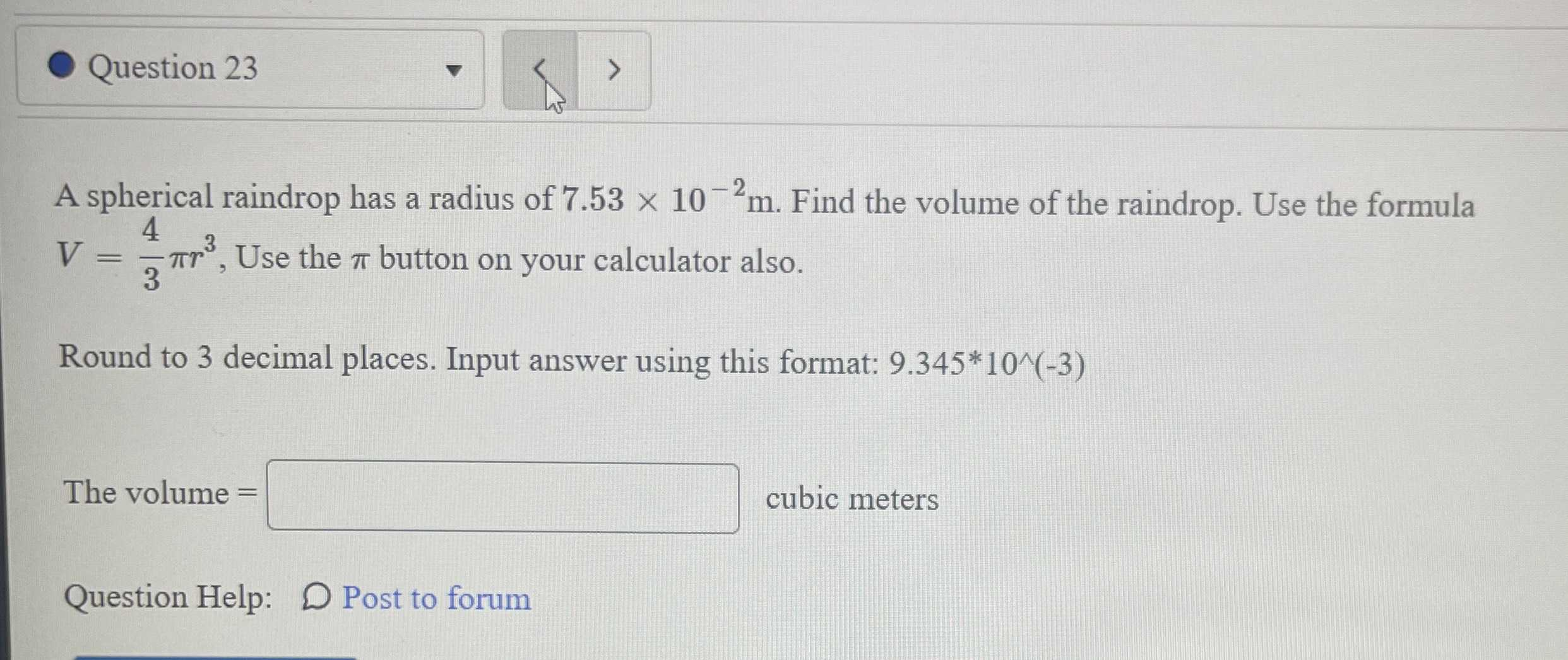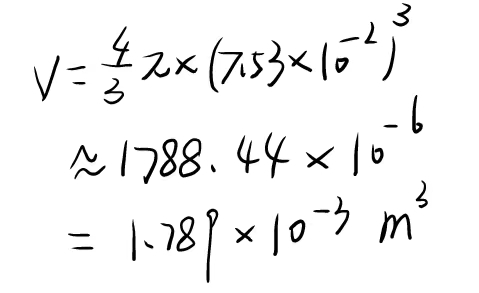### Still have math questions?

Algebra
QuestionQuestion $$23$$ A spherical raindrop has a radius of $$7.53 \times 10 ^ { - 2 } m$$ . Find the volume of the raindrop. Use the formula $$V = \frac { 4 } { 3 } \pi r ^ { 3 } ,$$ Use the $$\pi$$ button on your calculator also. Round to $$3$$ decimal places. Input answer using this format: $$9.345 ^ { * } 10 ^ { \wedge } ( - 3 )$$

The volume $$=$$ Cubic meters Question Help: Post to forum